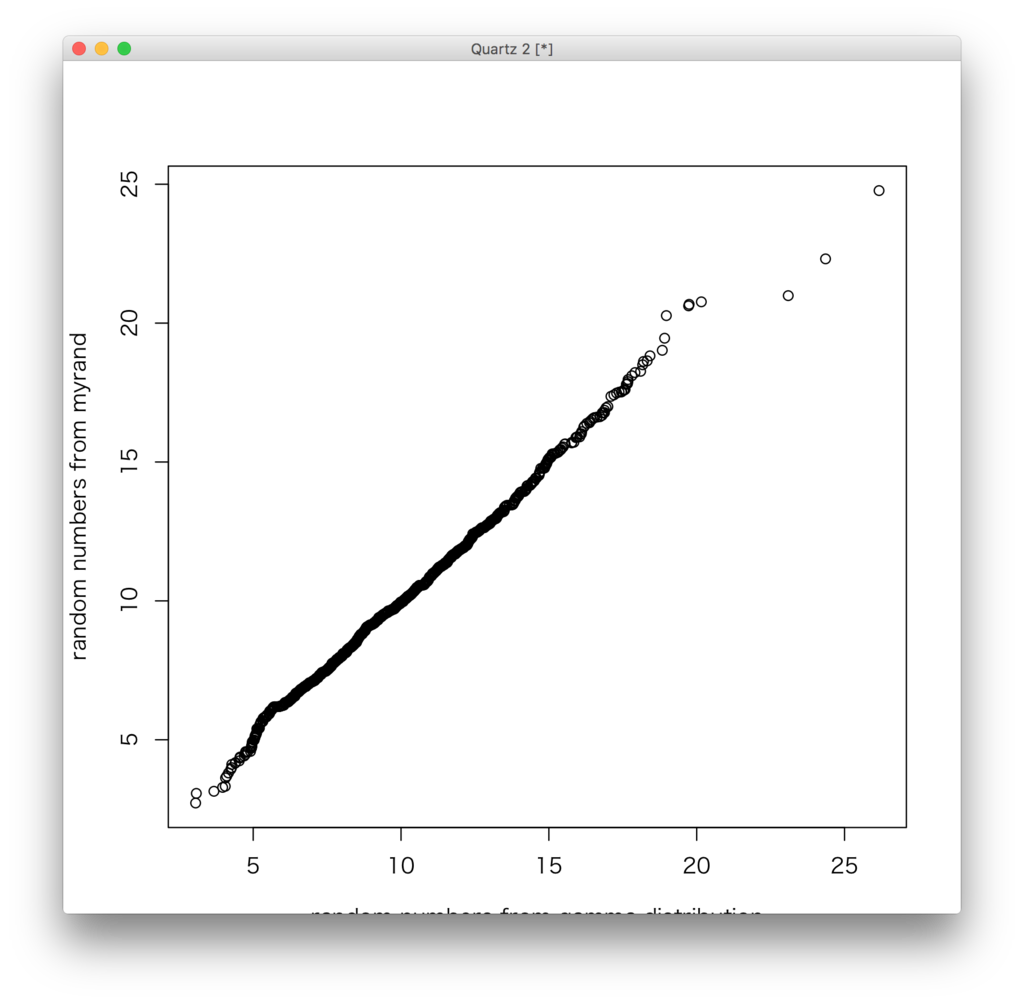# Example of Rejection Sampling

をやってみるてすと。PRMLの第11章サンプリング法のところのP242とかです。

Suppose we can not make the random samples which come from gamma distribution. Let us consider making them from the cauchy distribution. So proposal distribution is the cauchy distribution. The plot these distribution is as follows.

k <- 2.3
plot(function(x){dgamma(x,shape=10)},0,30,
ylab="",col="red",lwd=3)
title(main="棄却サンプリング")
legend(15,0.12,c("ガンマ関数","一般化されたコーシー分布"),lwd=3,col=c("red","blue"))In the above case, the constant valueis 2.3. For all x in the domain, the generalized cauchy distribution is greater than gamma distribution.

According to the マルコフ連鎖モンテカルロ法 (統計ライブラリー) in page 7-8, rejection sampling is written as,

Even if it is difficult to sample from the target distribution directly, we may get the random numbers from the target distribution indirectly using the distribution which we can sample easily. Such a method is called rejection sampling or accept-rejection sampling. Let.represents the probability density function and C is the normalizing constant term which makes sum ofbe equal to 1. When it is difficult to sample from, suppose that there is the distribution functionwhich can easily generate the random numbers, andhas the constant valuewhich satisfies. Under such assumptions, we can generate the random numbers fromindirectly as follows.

1. generate random numberfrom.
2. calculate ratio.
3. generate the uniform random numberfrom interval.
4. adoptif, otherwise return to 1.

And I implemented above algorithm with R. The program is as follows.

myrand <- function(){
x <- rcauchy(1,scale=5,location=10)
alpha <- dgamma(x,shape=10) / (k * dcauchy(x,scale=5,location=10))
u <- runif(1)
if(u < alpha){
return(x)
}else{
print("false")
myrand()
}
}

n <- 1000
qqplot(rgamma(n,shape=10),sapply(1:n,function(x){myrand()}),xlab="random numbers from gamma distribution",ylab="random numbers from myrand")The above qqplot seems to be linear, so I found my program went well.パターン認識と機械学習 下 (ベイズ理論による統計的予測)

• 作者: C.M.ビショップ,元田浩,栗田多喜夫,樋口知之,松本裕治,村田昇
• 出版社/メーカー: 丸善出版
• 発売日: 2012/02/29
• メディア: 単行本
• 購入: 6人 クリック: 14回
• この商品を含むブログを見る